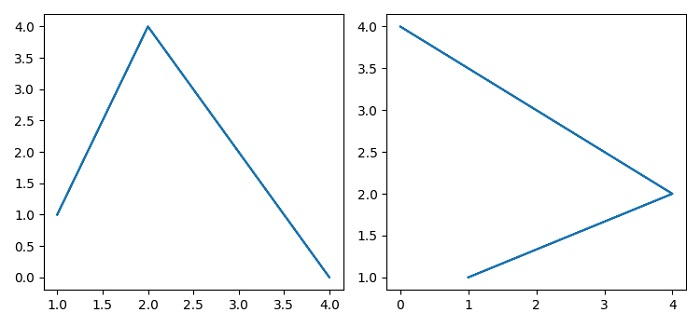# Setting active subplot using axes object in Matplotlib

To set active subplot axes object in matplotlib, we can use subplots() method to add the axes as a subplot arrangement.

## Steps

• Set the figure size and adjust the padding between and around the subplots.

• Create x and y lists for data points.

• Create a figure and a set of subplots using subplots() method with one row and two columns.

• Add an axes to the current figure and make it the current axes, with axis object at the 0th index.

• Plot x and y data points using plot() method.

• Add an axes to the current figure and make it the current axes, with axis object at the 1st index.

• Plot x and y data points using plot() method.

• To display the figure, use show() method.

## Example

import matplotlib.pyplot as plt

plt.rcParams["figure.figsize"] = [7.50, 3.50]
plt.rcParams["figure.autolayout"] = True

x = [1, 2, 4, 2, 1]
y = [1, 4, 0, 4, 1]

fig, axs = plt.subplots(1, 2)

plt.axes(axs)
plt.plot(x, y)

plt.axes(axs)
plt.plot(y, x)

plt.show()

## Output